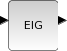Scilab Home page | Wiki | Bug tracker | Forge | Mailing list archives | ATOMS | File exchange
Change language to: Français - Português - 日本語

See the recommended documentation of this function

Scilab help >> Xcos > palettes > Matrix operation palette > MATEIG

# MATEIG

Matrix Eigenvalues

### Block Screenshot### Description

The MATEIG block computes the eigenvalues and the eigenvectors of a square input matrix U. When the decomposition type is set to:

• 1, the block outputs the eigenvalues in a vector form, if the input is a MxM matrix the output is a Mx1 vector.

• 2, the block outputs two matrices. For an MxM input matrix, the first output is a MxM diagonal matrix composed by the eigenvalues, and the second is a MxM matrix where columns are the eigenvectors.

The equivalent of the MATEIG block in Scilab is spec

### Dialog box• Datatype(1=real double 2=Complex)

It indicates the type of the output. It support only the two types double (1) and complex (2). If we input another entry in this label xcos will print the message "Datatype is not supported".

Properties : Type 'vec' of size 1.

• decomposition type (1=eig values 2=eig values+eig vectors

To select the form of the output.

Properties : Type 'vec' of size 1.

### Example

```A=[1 12 32;21 35 46;70 8 19]
When the "Decomposition type" is set to one the output is y=[-35.649;14.279;76.369]
When the "Decomposition type" is set to two the outputs are:
y1=[-35.649 0.000 0.000;0.000 14.279 0.000;0.000 0.000 76.369]
y2=[0.557 0.080 0.349;0.330 -0.922 0.770;-0.762 0.379 0.533]```

### Default properties

• always active: no

• direct-feedthrough: yes

• zero-crossing: no

• mode: no

• regular inputs:

- port 1 : size [-1,-1] / type 1

• regular outputs:

- port 1 : size [-1,1] / type 2

• number/sizes of activation inputs: 0

• number/sizes of activation outputs: 0

• continuous-time state: no

• discrete-time state: no

• object discrete-time state: no

• name of computational function: mat_vps

### Interfacing function

• SCI/modules/scicos_blocks/macros/MatrixOp/MATEIG.sci

### Computational function

• SCI/modules/scicos_blocks/src/c/mat_vps.c

• SCI/modules/scicos_blocks/src/c/mat_vpv.c

• SCI/modules/scicos_blocks/src/c/matz_vps.c

• SCI/modules/scicos_blocks/src/c/matz_vpv.c